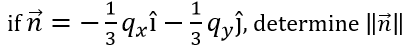# Scalar multiplication of vectors

### Scalar multiplication of vectors

We have learnt that for a vector arrow, the greater the length, the greater the magnitude. Now what if we somehow want to increase or decrease the magnitude of an existing vector? In this section, we will introduce scalar multiplication – a tool that allows us to lengthen or shorten a vector arrow, in other words, a technique that alters the magnitude of a vector.

#### Lessons

• 1.
Given that vector $\vec{v}=$<5,3> , determine $6\vec{v}$

• 2.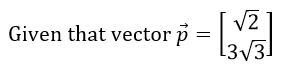a)
determine $0\vec{p}$

b)
find the magnitude of $0\vec{p}$

• 3.
Given that vector $\vec{w} =$<$10,-4$>
a)
determine $\frac{1}{2}\vec{w}$

b)
find the magnitude of $\frac{1}{2}\vec{w}$

• 4.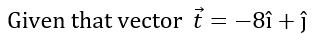a)
determine $3\vec{t}$ and $||3t||$

b)
determine $-3\vec{t}$ and $||-3t||$

• 5.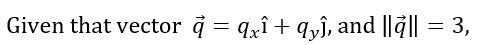a)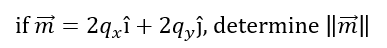b)